# Long and wide

What is the area of a rectangle that is 1 1/4 meters long and 3/4​ of a meter wide

S =  0.9375 m2

### Step-by-step explanation: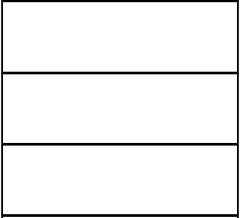Did you find an error or inaccuracy? Feel free to write us. Thank you!Tips to related online calculators
Need help to calculate sum, simplify or multiply fractions? Try our fraction calculator.

#### You need to know the following knowledge to solve this word math problem:

We encourage you to watch this tutorial video on this math problem:

## Related math problems and questions:

• Mason 3Mason has a rectangular garden that is 2/3 yard wide by 7/4 yards long. What is the area of Mason’s garden? Use a drawing to show your work.
• A rectangle 6A rectangle measures 2 4/5 inches by 2 1/5 inches. What is its area?
• Juila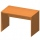Juila has a rectangular table that is 3 1/2 feet long and 1 3/4 feet wide. What is the area of Juila's table?
• Area and perimeter 2Find the area and the perimeter of a rectangle of length 45 1/2 cm and breadth 16 2/3 cm.
• Living roomMarina's new living room is a rectangle with a length of 8 meters and a width of 5 meters. She wants to buy a carpet to cover half of the living room and each square meter of the carpet has a cost of \$4. How much will be the total cost for Marina?
• Bathroom 2A bathroom is 2.4 meters long and 1.8 meters wide. How many square tiles 1 dm on each side are to be used to cover it?
• In the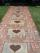In the garden, workers will pave a 1-meter-wide sidewalk with tiles around the block-shaped pool. The dimensions of the bottom of the pool are 8.5 meters and 6 meters. The height of the pool walls is 2 meters. How many m2 of pavement will be laid with til
• How many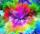How many cans of blue paint need to be bought if the interior of the garden pool, which is 5 m long, 3 m wide and 1 m deep, is to be painted? There is 1 kg of paint in each can. One can is enough for 8 m2 of area.
• AlexandraAlexandra made a rectangular quilt the measured 3 1/4. 2 3/4 feet in width. What is the area of the quilt in square feet? Write an equation to solve.
• Wall painting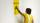The wall is 4 meters wide and 2 meters high. The window in the wall has dimensions of 2x1,8 meters. How many liters of color is needed to paint two-layer these walls, if the 1 m2 needs 1 liters of paint?
• Three rectangles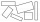Some wire is used to make 3 rectangles: A, B, and C. Rectangle B's dimensions are 3/5 cm larger than Rectangle A's dimensions, and Rectangle C's dimensions are again 3/5 cm larger than Rectangle B's dimensions. Rectangle A is 2 cm by 3 1/5 cm. What is the
• Swimming pool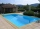A swimming pool 30 meters long is filled with water to a depth of 1 meter at the shallow end, and 5 meters at the deep end and abcd the vertical area of the pool has the shape of a trapezium with the area given by S(abcd)= 1/2 (ab + cd) x ad. What is the
• Father's gardenJohn's father has a garden in the back yard. The dimensions of the garden are shown here. A green rectangle is shown. The width is labeled as 2 and two-thirds feet. The length is labeled as 7 and three-fourths feet. Calculate the area of the garden. You f
• The length 5The length and breadth of rectangular field is 3 1/5 m  and 1 3/7 m. Find the area of rectangular field.
• RainfallHow many liters of water did fell in a 32m long and 8m wide garden, if 8mm of rain fell?
• Jewelry box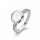The bottom of Zeyda's jewelry box is a rectangle with the length of 5 3/8 inches and a width of 3 1/4 inches. What is the area of the bottom of the jewelry box?
• Decorative fenceThe rectangular garden is 57 m long and 42 m wide. Calculate how much m² it will reduce if it encloses with a 60 cm wide decorative fence.